### Home > PC > Chapter 6 > Lesson 6.2.3 > Problem6-97

6-97.
1. Find the period of each function. Homework Help ✎

1. y = 5 sin 3x

2. y = cos πx

3. y = sin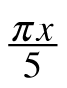4. y = cos+ 3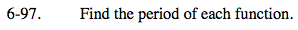Use the formula:
pb = 2π

p = period
b = frequency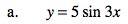3 is the frequency.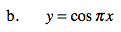π is the frequency.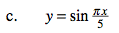${\frac{\pi}{5}\text{ is the frequency.}}$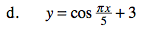${\frac{\pi}{5}\text{ is the frequency.}}$